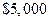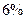Chapter 17, Problem 6SCQ### Principles of Economics 2e

2nd Edition
Steven A. Greenlaw; David Shapiro
ISBN: 9781947172364

#### Solutions

Chapter
Section### Principles of Economics 2e

2nd Edition
Steven A. Greenlaw; David Shapiro
ISBN: 9781947172364
Textbook Problem

# What is the total amount of interest from aloan after three years with a simple interest rate ofTo determine

To Calculate: the total amount of interest from a loan.

Explanation

The formula for simple interest is given as:

A = P(1+rt)

Where, A = Total Accrued Amount

P= Principal Amount

r = Rate of interest

t = Time period

A=5,000{1+0

### Still sussing out bartleby?

Check out a sample textbook solution.

See a sample solution

#### The Solution to Your Study Problems

Bartleby provides explanations to thousands of textbook problems written by our experts, many with advanced degrees!

Get Started